knn深度学习_knn分类 knn - CSDN
• 先看一个实例来体会一下什么叫做KNN。已知一堆数据a和一堆数据0，然后有一个未知数据c，要判断c到底属于a还是0.如果是1NN,就找到离C最近的数据，离哪一个最近，就判断c属于哪一类。如果利用3NN，如下图所示，以c为...
先看一个实例来体会一下什么叫做KNN。已知一堆数据a和一堆数据0，然后有一个未知数据c，要判断c到底属于a还是0.如果是1NN,就找到离C最近的数据，离哪一个最近，就判断c属于哪一类。如果利用3NN，如下图所示，以c为半径画圆，直到找出三个数为止，分别有一个a，两个0，则说明c属于0.（注意K一定是奇数可以保证不会平票）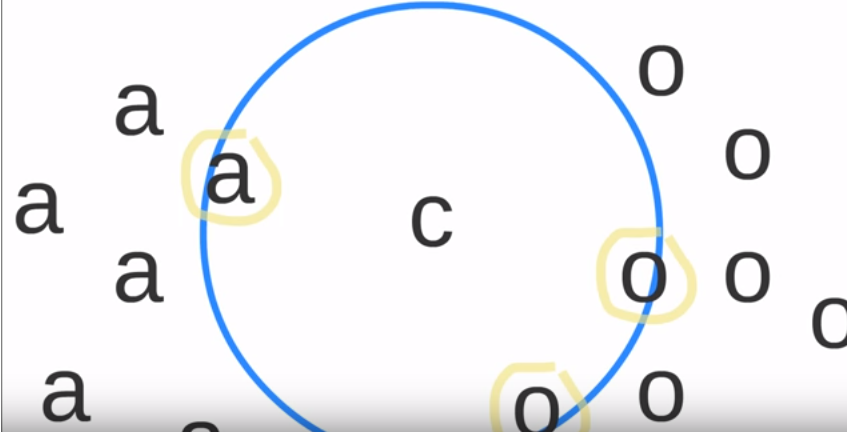接下来通过一个实例来讲一下KNN

分类问题：

一个学生是不是三好学生通过德智体三个方面来进行评价。如果我们的样本空间如下：（所谓样本空间，又叫Training Data，也就是用于机器学习的数据）

学生编号
向量1
向量2
向量3
是否为三好学生
赵1
9
8
9
是
钱2
8
7
8
是
孙3
4
6
7
不是
李4
3
5
9
不是
周5
9
3
9
是
吴6
2
9
7
？

那么吴6是三好学生不是呢？我们使用KNN算法来判断。假设K=3，K应该是一个奇数，这样可以保证不会有平票，下面是我们计算（2，9,7）到所有点的距离。（距离公式，可以参看K-Means算法中的一些距离定义）

学生编号
向量1
向量2
向量3
到吴六的距离
是否为三好学生
赵一
9
8
9

是
钱二
8
7
8

是
孙三
4
6
7

不是
李四
3
5
9

不是
周五
9
3
9

是

离吴六最近的几个人是孙三，李四，钱二，只有钱儿是三好学生，孙三和李四都不是，那么吴六也不是三好学生了，这样就可以把吴六分类为不是三好学生。

当然利用kNN也可以解决回归问题，但是最大最有效的就是分类问题了。2）对于KNN算法中找到离自己最近的K个点需要使用到最大堆数据结构。也会做出阐述。

接下来先通过一个实例来使用KNN对MNIST最经典的字符识别做一下分类实验。

代码如下：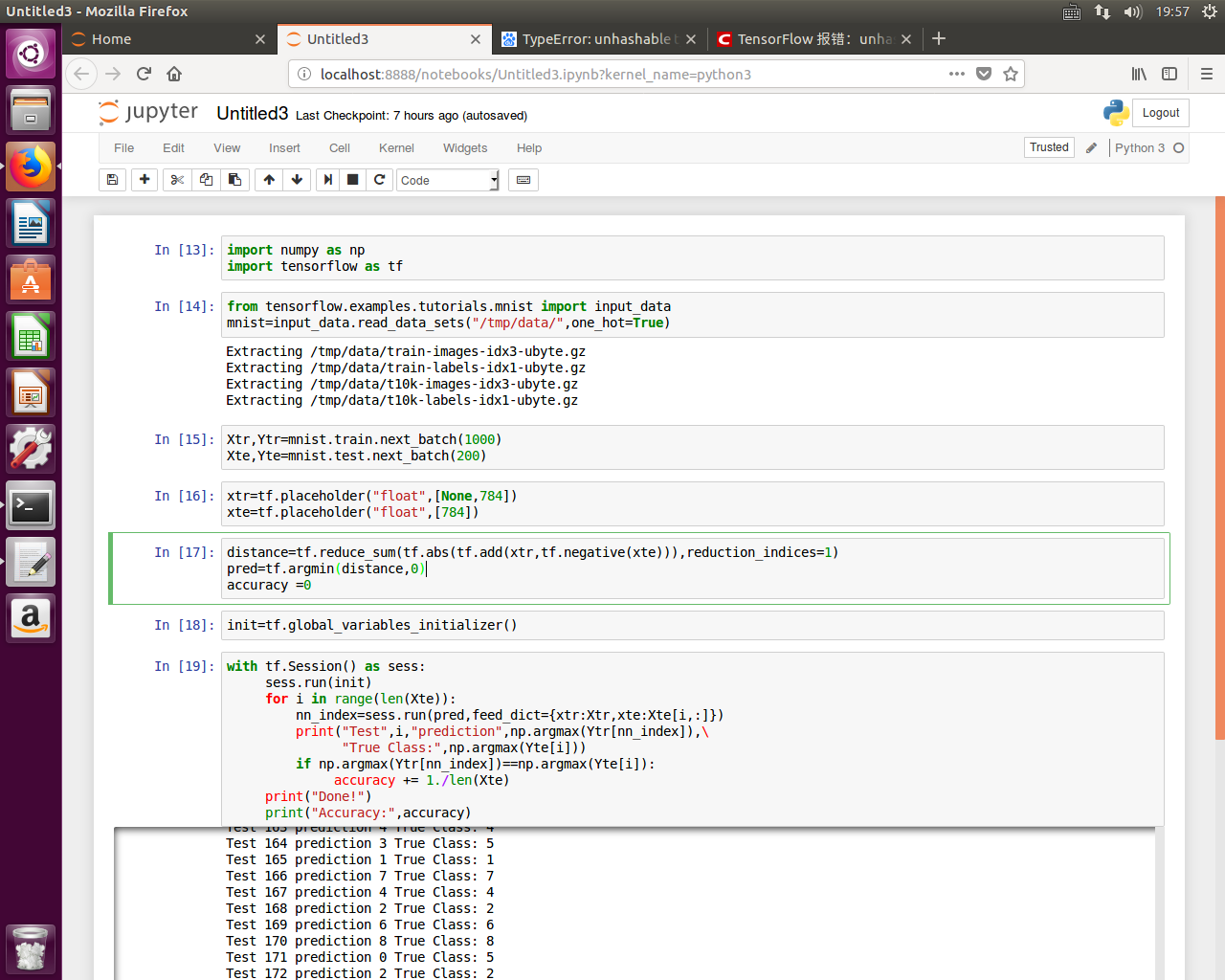程序说明：1.因为使用的为one_hot编码，故Ytr为n*10的数组，每个数组形式都为【九个0+一个1】的形式，找到Ytr【nn_index】后只需要使用np.argmax()函数找到1所在位置即为数字。

补充：关于placeholder与reduce_sum的一些问题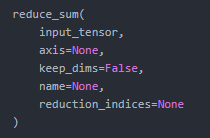说明：
reduce_sum() 就是求和，由于求和的对象是tensor，所以是沿着tensor的某些维度求和。函数名中加了reduce是表示求和后会降维，当然可以通过设置参数来保证不降维，但是默认就是要降维的。

参数解释：
1）input_tensor：输入的张量。
2）axis：沿着哪个维度求和。
对于二维的input_tensor张量，0表示按列求和，1表示按行求和，[0, 1]表示先按列求和再按行求和。
3）keep_dims：默认值为Flase，表示默认要降维。若设为True，则不降维。
4）name：名字。
5）reduction_indices：默认值是None，即把input_tensor降到 0维，也就是一个数。
对于2维input_tensor，reduction_indices=0时，按列；reduction_indices=1时，按行。
注意，reduction_indices与axis不能同时设置。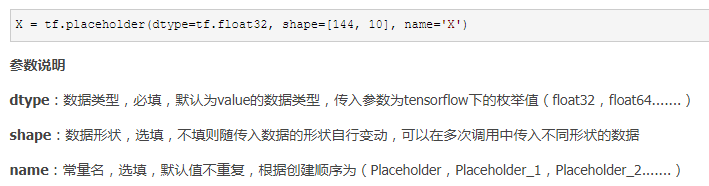其中当shape为三维矢量，比如[2,2,3]

后两维表示矩阵为[2,3]格式的，分为两层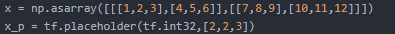那么数据格式是这样的。运用在reduce_sum中，如果axis=0，则第一维求和。即上下数分别对应求和。如果axis=1，则第二维求和，即第一行+第二行，求出结果是一行三列。axis=2.则第三维求和，即第一列+第二列+第三列。结果是两行一列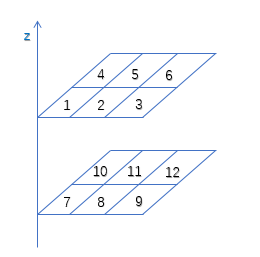展开全文• 1，上午继续看书，主要看了深度学习的发展历程以及主要技术介绍 2，中午思考了决策树算法中的数据准备阶的矩阵装换，将类似Excel数据表格的形式数据转换为0-1矩阵  数据大致格式为：表头为特征向量名称以及输出...
总结一下今天的学习过程
1，上午继续看书，主要看了深度学习的发展历程以及主要技术介绍
2，中午思考了决策树算法中的数据准备阶的矩阵装换，将类似Excel数据表格的形式数据转换为0-1矩阵
数据大致格式为：表头为特征向量名称以及输出标签名称，而特征向量的值又是多个，决策树中是将每一个特征向量多维化，形成一个0-1矩阵
将每一个特征向量多维化
本想着使用java实现，结果字典格式的数据在Java中表示比较麻烦，就没有真实实现，这里说一下思路
2，遍历list再遍历其中的treemap，并赋值到一维数组中
3，创建一个二维数组用来表示装换的矩阵，遍历Excel表，根据每一行的数据遍历值获取对应的一维数组中的下标m，将M以前为赋值的二维数组中的一行数据，m前面的为0，后面的为1，一次类推，得出转换的二维矩阵

3，下午看了KNN，学习了基本原理以及并敲写了代码，做了实现
使用了Python库中函数，根据网上教程也敲写了业务逻辑真实实现代码，由于思想以及原理比较客观直接，这里就不赘述了
4，晚上观看了svm支持向量积，了解了基本原理，
但是其中的数学逻辑装换还是比较蒙圈，，，
看了代码的基本实现，本地还没装配环境，如numpy，pylab等，希望明天能顺利安装

加油，美好的一天


展开全文•   在学习深度和图像识别的时候，看见了一个比较有意思的算法——KNN算法，该算法是图像分类中最简单的算法之一。 基础理论   KNN算法全称是K-最近邻算法，英文名称是K-NearestNeighbor，简称为KNN；从算法名称上...
k-最近邻算法基础理论欧式距离（Euclidean distance）曼哈顿距离（Manhattan distance）代码实例
在学习深度和图像识别的时候，看见了一个比较有意思的算法——KNN算法，该算法是图像分类中最简单的算法之一。
基础理论
KNN算法全称是K-最近邻算法，英文名称是K-NearestNeighbor，简称为KNN；从算法名称上，可以猜出，是找到最近的k个邻居，在选取到的k个样本中选取出最近的且占比最高的类别作为预测类别。如下图所示：KNN算法
上图所指示，蓝色的正方形和红色的三角形是已经存在的样本，而绿色的圆（待测样本）是要被赋予为那种类别，即是红色的三角形还是蓝色的正方形。如果取$k=3$，通过上图可以看出，红色的三角形占比为$\frac{2}3$，蓝色正方形占比为$\frac{1}3$，因为红色三角形的占比大于蓝色正方形的占比，所以绿色的圆将被赋予为红色三角形的类别。如果取$k=5$，通过上图可以看出，红色的三角形占比为$\frac{2}5$，蓝色正方形占比为$\frac{3}5$，因为红色三角形的占比小于蓝色正方形的占比，所以绿色的圆将被赋予为蓝色正方形的类别。
KNN算法的计算逻辑总结：

给定测试对象，计算它与训练集中每个对象的距离；
确定最近的k个训练对象，作为测试对象的邻居；、
根据这k个训练对象所属的类别，找到占比最高的类别作为测试对象的预测类别。

在KNN算法中，你会发现影像KNN算法准确度的因素主要有两个：一是计算测试对象与训练集中各个对象间的距离；二是k的个数的选择。
主要有两种距离计算方式：曼哈顿距离和欧式距离
欧式距离（Euclidean distance）
$d(x,y) =\sqrt{(x_1-y_1)^2 + (x_2-y_2)^2 + (x_3-y_3)^2 + ... + (x_n-y_n)^2} =\sqrt\sum^{n}_{i=1}{(x_i-y_i)^2}}$
缺点：它将样本的不同属性（即各指标或各变量量纲）之间的差别等同看待，这一点有时不能满足实际要求。比如年龄和学历对工资的影响，将年龄和学历同等看待；收入单位为元和收入单位为万元同等看待。
标准化欧式距离，将属性进行标准化处理，区间设置在[0,1]之间，减少量纲的影响
曼哈顿距离（Manhattan distance）
$d_{12} =|x_{11}-x_{21}| + |x_{12}-x_{22}| + |x_{13}-x_{24}| + ... + |x_{1k}-x_{2k}| =\displaystyle \sum^{}_{k=1}{|x_{1k}-x_{2k}|}$
代码实例
import matplotlib.pyplot as plt
import numpy as np
import operator

def KNN_distance(k, dis, trains_1, labels_2, test):

#曼哈顿距离（Manhattan distance）： 简称M
#欧式距离（Euclidean Metric）： 简称E
assert  dis == 'M' or dis == 'E'
count = test.shape
label_list = []

# 欧式距离 sqrt((x1-x2)^2 + (y1-y2)^2)
if dis == 'E':
for i in range(count):
distance = np.sqrt(np.sum(((trains_1 - np.tile(test[i], (trains_1.shape, 1))) ** 2), axis=1))
nearest_k = np.argsort(distance)
topK = nearest_k[:k]
classCount = {}
for i in topK:
classCount[labels_2[i]] = classCount.get(labels_2[i], 0) + 1
sortedClassCount = sorted(classCount.items(), key=operator.itemgetter(1), reverse=True)
label_list.append(sortedClassCount)
# 曼哈顿距离：|x1-x2| + |y1-y2|
elif dis == 'M':
for i in range(count):
distance = np.sum(np.abs(trains_1 - np.tile(test[i], (trains_1.shape, 1))), axis=1)

nearest_k = np.argsort(distance)
topK = nearest_k[:k]
classCount = {}
for i in topK:
classCount[labels_2[i]] = classCount.get(labels_2[i], 0) + 1
sortedClassCount = sorted(classCount.items(), key=operator.itemgetter(1), reverse=True)
label_list.append(sortedClassCount)
return label_list

def CreatDataSet():
# group = np.array([[0.2, 1], [0.3, 4], [0.4, 2], [1, 0.2], [3, 0.5], [5, 0.7]])
group = np.array([[1.0, 2.0], [1.2, 0.1], [0.1, 1.4], [0.3, 3.5], [1.1, 1.0], [0.5, 1.5]])
labels = np.array(['A', 'A', 'B', 'B', 'A', 'B'])
return (group, labels)

if __name__ == '__main__':
group, labels = CreatDataSet()
# 绘制点在坐标系中的位置
# plt.scatter(group[labels == 'A', 0], group[labels == 'A', 1], color='r', marker='*')
# plt.scatter(group[labels == 'B', 0], group[labels == 'B', 1], color='g', marker='+')
# plt.show()

# 欧氏距离
y_test_pred = KNN_distance(2, 'E', group, labels, np.array([[1.0, 2.1], [0.4, 2.0]]))
print(y_test_pred)
print("****************************")
# 曼哈顿距离
y_test_pred = KNN_distance(2, 'M', group, labels, np.array([[1.0, 2.1], [0.4, 2.0]]))
print(y_test_pred)
# output：
#     ['A', 'B']
#     ****************************
#     ['A', 'A']

从输出结果上看，两次的输出结果是不同的，正常情况下应该输出一致， 下面会有一个简单的分析，若有想深入了解的，可以深入研究，
欧式距离： $\sqrt{(0.6)^2 + (0)^2}$ 和$\sqrt{(0.5)^2 + (0.1)^2}$ 是不相同的
曼哈顿距离：$|0.6 + 0|$ 和$|0.5 + 0.1|$ 是相同的


展开全文深度学习 神经网络 机器学习 自动驾驶 自然语言处理
• 在几经摸索下，终于用python开始写下了自己的第一个机器学习算法KNN 这是一个分类算法，可以通过已有标签，判断一个输入的事物是不是已知的标签，其实这个算法算是入门级的，用来练手刚刚好 写完以后觉得，机器...
在几经摸索下，终于用python开始写下了自己的第一个机器学习算法KNN
这是一个分类算法，可以通过已有标签，判断一个输入的事物是不是已知的标签，其实这个算法算是入门级的，用来练手刚刚好
写完以后觉得，机器学习比较麻烦的是处理数据，输入数据，困难的是对于数据的建模，代码其实写不来多少
话不多说附上我的核心源码
test.py

def classify0(inX,dataSet,lables,k):
dataSetSize = dataSet.shape

diffMat = tile(inX,(dataSetSize,1)) - dataSet
sqDiffMat = diffMat **2
sqDistances = sqDiffMat.sum(axis=1)
distances = sqDistances ** 0.5
sortedDisIndicies = distances.argsort()
classCount = {}
for i in range(k):
voteIlabel = lables[sortedDisIndicies[i]]
classCount[voteIlabel] = classCount.get(voteIlabel,0)+1

sortedClassCount = sorted(classCount.iteritems(),key = operator.itemgetter(1),reverse=True)
return sortedClassCount

这是knn核心分类代码，所有一切的基础都是在此基础上得到的

还有一个我抄写大神写的 利用机器学习识别0-9图片识别的源码

from numpy import *
from os import listdir
import test

def hand_writing_class_test():
hwlabels = []

training_file_list = listdir('digits/trainingDigits')
m = len(training_file_list)
training_mat = zeros((m,1024))
for i in range(m):
file_name_str = training_file_list[i]
file_str = file_name_str.split('.')
class_num_str = int(file_str.split('_'))
hwlabels.append(class_num_str)
training_mat[i,:] = img_vector('digits/trainingDigits/%s'%file_name_str)
test_file_list = listdir('digits/testDigits')
error_count = 0.0
m_test = len(test_file_list)
for i in range(m_test):
file_name_str = test_file_list[i]
file_str = file_name_str.split('.')
class_num_str = int(file_str.split('_'))
vector_under_test = img_vector('digits/testDigits/%s'%file_name_str)
class_ifier_results = test.classify0(vector_under_test,training_mat,hwlabels,3)
print ("the class_ifier_results came back with:%d,the real answer is %d"%(class_ifier_results,class_num_str))
if(class_ifier_results!=class_num_str):error_count +=1.0
print "\n the total number of errors is %d" %error_count
print "\n the total error rate is %f" %(error_count/float(m_test))

暂时先写着么多，我以后一点一点的学习，一点一点的写


展开全文深度学习 算法
• 之前为了熟悉机器学习的东西去搞kaggle的东西，然后就从Titanic入门咯，结果发现并没有对机器学习的东西有深入的理解，做数据挖掘的时候直接调用sklearn里面的框架，根本不用去想机器学习的公式的运用和基础的实现，...
    之前为了熟悉机器学习的东西去搞kaggle的东西，然后就从Titanic入门咯，结果发现并没有对机器学习的东西有深入的理解，做数据挖掘的时候直接调用sklearn里面的框架，根本不用去想机器学习的公式的运用和基础的实现，想用SVM就直接from sklearn import svm，然后clf = svm.SVC(kernel='linear', C=1)往这里面填几个参数，知道基本原理和参数的含义就行。直到我看到这段话：很多人说深度学习就是个黑箱子，把图像预处理之后丢进 tensorflow 就能出来预测结果，简单有效又省时省力。但正如我在上一篇推送中所说，如果你已是一名功力纯厚的深度学习工程师，这么做当然没问题。但我想大多数人也和我一样，都是走在学习深度学习的路上，一上来就上框架并没有什么特别不妥之处，但总归是对你理解深度学习的黑箱机制是了无裨益的。所以，我建议在学习深度学习的路上，从最简单的感知机开始写起，一步一步捋清神经网络的结构，以至于激活函数怎么写、采用何种损失函数、前向传播怎么写、后向传播又怎么写，权值如何迭代更新，都需要你自己去实现。若在一开始就直接调用框架，小的 demo 可以跑起来，糊弄一时，看起来就像是鸠摩智在内力未到的情形下强行练习少林寺的 72 绝技，最后走火入魔。我发现我好像走火入魔了，这时我想着我又该把基础打打牢了，之前看过吴老师的机器学习，只能算是理论上有了一定认识，没做过多实践，代码和理论还没好好联系起来，然后就找到了李飞飞深度学习与计算机视觉，下面开始我的第一课——KNN。先贴点网址  https://zhuanlan.zhihu.com/p/21930884     大牛搞的CS231n官方笔记授权翻译总集http://cs231n.stanford.edu/2016/syllabus    英文的CS231n官方笔记http://www.cs.toronto.edu/~kriz/cifar.html    做学习的数据集特别感谢翻译笔记的哥们。第一部分 KNN算法实现先贴原理，具体原理直接看笔记，我自己做个总结一、概念&意义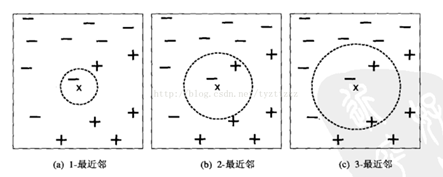二、计算步骤：1、算距离：给定测试对象，计算它与训练对象距离2、找邻居：圈定距离最近的 k个对象，作为测试对象的近邻3、做分类：根据这k个近邻归属的主要类别，来对测试对象分类三、三个基本要素I、距离度量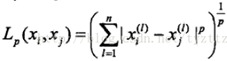a)当p=2时，为欧式距离：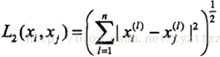b)当p=1时，为曼哈顿距离：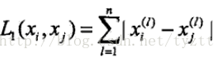II、K值的选择K值越小，整体模型越复杂，容易发生过拟合K值越大，整体模型越简单，近似误差会增大（误分类）III、测试对象类别的判定a)   多数表决：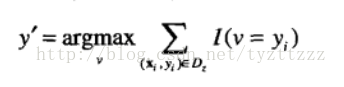其中v是类标号，yi是一个最近邻的类标号，I(.)是指示函数，如果其参数为真，则返回1，否则，返回0b)   距离加权表决：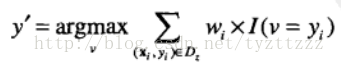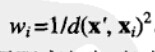# 损失函数的公式L = -(1/N)∑i∑j1(k=yi)log(exp(fk)/∑j exp(fj)) +λR(W) 再上代码import numpy as npclass KNearestNeighbor(object):#首先是定义一个处理KNN的类  """ a kNN classifier with L2 distance """   def __init__(self):      pass   def train(self, X, y):    self.X_train = X    self.y_train = y       def predict(self, X, k=1, num_loops=0):    if num_loops == 0:      dists = self.compute_distances_no_loops(X)    elif num_loops == 1:      dists = self.compute_distances_one_loop(X)    elif num_loops == 2:      dists = self.compute_distances_two_loops(X)    else:      raise ValueError('Invalid value %d for num_loops' % num_loops)     return self.predict_labels(dists, k=k)   def compute_distances_two_loops(self, X):    num_test = X.shape    num_train = self.X_train.shape    dists = np.zeros((num_test, num_train))    for i in xrange(num_test):      for j in xrange(num_train):        dists[i][j] = np.sqrt(np.sum(np.square(self.X_train[j,:] - X[i,:])))    return dists   def compute_distances_one_loop(self, X):    num_test = X.shape    num_train = self.X_train.shape    dists = np.zeros((num_test, num_train))    for i in range(num_test):   #i就是类别，i=1即类1      dists[i,:] = np.sqrt(np.sum(np.square(self.X_train-X[i,:]),axis = 1))    return dists   def compute_distances_no_loops(self, X):    num_test = X.shape    num_train = self.X_train.shape    dists = np.zeros((num_test, num_train))   #dists(测试类别数，测试集的数量)    dists = np.multiply(np.dot(X,self.X_train.T),-2)      sq1 = np.sum(np.square(X),axis=1,keepdims = True)      sq2 = np.sum(np.square(self.X_train),axis=1)      dists = np.add(dists,sq1)      dists = np.add(dists,sq2)      dists = np.sqrt(dists)     return dists  def predict_labels(self, dists, k=1):    num_test = dists.shape    y_pred = np.zeros(num_test)    for i in range(num_test):      closest_y = []        closest_y = self.y_train[np.argsort(dists[i,:])[:k]]        y_pred[i]=np.argmax(np.bincount(closest_y))            return y_pred代码总结python自己基本上算是没学过，靠着C++的底子强行直接上了，给自己留一点提示吧def __init__(self):      pass   #一般不pass，一般def __init__(self,X,y):self.X=X，就是把外部传入的变量变成类内部的东西，当然也可以像代码中再搞个方法来做这个步骤def train(self, X, y):    self.X_train = X    self.y_train = y这段代码就相当于做了刚才的工作    if num_loops == 0:      dists = self.compute_distances_no_loops(X)    elif num_loops == 1:      dists = self.compute_distances_one_loop(X)    elif num_loops == 2:      dists = self.compute_distances_two_loops(X)这就是三种计算测试集中每张图片和训练集中每张图片的距离的方法，循环次数不同。计算之后返回dists如果是2*2的图片的话，dists=num_test_picture*(num_train_picture个test_picture与train_picture之间的距离)np.argsort(dists[i,:])对每一种num_test_picture对应的num_train_picture个距离进行排序closest_y = self.y_train[np.argsort(dists[i,:])[:k]]  然后对每一种num_test_picture取前k个到closest_y，closest_yp.argmax:最大值的索引x = np.array([0, 1, 1, 3, 2, 1, 7])  索引0出现了1次，索引1出现了3次......索引5出现了0次...... np.bincount(x)，因此，输出结果为：array([1, 3,1, 1, 0, 0, 0, 1])y_pred[i] = np.argmax(np.bincount(closest_y))#就把索引出现最多的那个所以赋值到y_pred[i]第二部分 导入数据集和做预测# -*- coding: utf-8 -*-import pickle as pimport numpy as npimport osimport KNearestNeighbordef load_CIFAR_batch(filename):    """ 载入cifar数据集的一个batch """    with open(filename, 'rb') as f:        datadict = p.load(f, encoding='latin1')        X = datadict['data']        Y = datadict['labels']        X = X.reshape(10000, 3, 32, 32).transpose(0, 2, 3, 1).astype("float")        Y = np.array(Y)        return X, Ydef load_CIFAR10(ROOT):    """ 载入cifar全部数据 """    xs = []    ys = []    for b in range(1, 2):        f = os.path.join(ROOT, 'data_batch_%d' % (b,))        X, Y = load_CIFAR_batch(f)        xs.append(X)         #将所有batch整合起来        ys.append(Y)    Xtr = np.concatenate(xs) #使变成行向量,最终Xtr的尺寸为(50000,32*32*3)    Ytr = np.concatenate(ys)    del X, Y    Xte, Yte = load_CIFAR_batch(os.path.join(ROOT, 'test_batch'))    return Xtr, Ytr, Xte, Yte    import numpy as npimport matplotlib.pyplot as pltplt.rcParams['figure.figsize'] = (10.0, 8.0)plt.rcParams['image.interpolation'] = 'nearest'plt.rcParams['image.cmap'] = 'gray'# 载入CIFAR-10数据集cifar10_dir = 'julyedu/datasets/cifar-10-batches-py'X_train, y_train, X_test, y_test = load_CIFAR10(cifar10_dir)这样算是导入了X_train=np.reshape(X_train,(X_train.shape,-1))X_test=np.reshape(X_test,(X_test.shape,-1))把X_train变成行为训练集图片张数，列为单张图片的各个像素点的各个通道，如果X_train有500张图，32*32大小，彩色的图像，那X_train就变成了500*(32*32*3)的矩阵X_train=X_train[0:10000,:]X_test=X_test[0:500,:]y_train=y_train[0:10000]y_test=y_test[0:500]然后训练集10000张，测试集取500来测试得把之前写的KNN算法的代码导入一下，KNN算法也是我写成了KNearestNeighbor.py，那就import KNearestNeighbor，导入完就可以用算法来做预测了，分别取k为不同的数，看看预测的准确率for k in [1, 3, 5, 10, 20, 50, 100]:  # use a particular value of k and evaluation on validation data  nn = KNearestNeighbor.KNearestNeighbor() #这里有两个KNearestNeighbor，一个是文件，后面的是文件里的类  nn.train(X_train, y_train)  # here we assume a modified NearestNeighbor class that can take a k as input  Yval_predict = nn.predict(X_test, k = k)  acc = np.mean(Yval_predict == y_test)  print( 'accuracy: %f' % (acc,))贴一下预测结果accuracy: 0.276000accuracy: 0.304000accuracy: 0.324000accuracy: 0.306000accuracy: 0.298000accuracy: 0.264000accuracy: 0.262000结果显示k=5的时候，准确率最高，至此为止，第一步算是成功的跨出去了，花了我好几天时间，，主要是python的api都不太熟悉。
展开全文• 参考：https://blog.csdn.net/xundh/article/details/73611249 包括算法解释、代码和数据集。
• 2、KNN 算法的核心思想是如果一个样本在特征空间中的k个最相邻的样本中的大多数属于某一个类别，则该样本也属于这个类别，并具有这个类别上样本的特性。 3、类别决策时，只与极少量的相邻样本有关。 4、距离的测量...深度学习
• 图像分类问题这是很久以前就引起关注的一类图像相关问题。 对于一张输入的图片，要判定它属于给定的一些标签/类别中的哪一个。看似很简单的一个问题，这么多年却一直是计算机视觉的一个核心问题。...计算机视觉 深度学习 图片分类
• KNN 分类的计算复杂度和训练集中的文档数目成正比，也就是说，如果训练集中文档总数为n，那么KNN 的分类时间复杂度为O(n)。 基本原理 基于统计的方法 来进行样本点分类判别 对于未知类别属性数据集中的点：...
• 学习KNN（一） 图像分类与KNN原理 学习KNN（二）KNN算法手写数字识别的OpenCV实现 学习KNN（三）KNN+HOG实现手写数字识别 简介 KNN算法，即K近邻算法是一种监督学习算法，本质上是要在给定的训练样本中找到与...机器学习 图像分类
• 本章背景 本章是来源于coursera课程 python-machine-learning中的作业1内容。 本章参考 Pandas—汇总和频数统计 Pandas—DataFrame切片 Pandas—数据结构之Series ...K最近邻 (KNN，k-NearestNe...
• 先来看深度学习的代码： # 由于发现使用KNN算法对于facebook地区的分类实在是缓慢 # 因此尝试使用深度学习的方法 import tensorflow as tf import numpy as np import pandas as pd from sklearn.model_selection ...深度学习 facebook
• 这是一个使用knn把特征向量进行分类的demo。 Knn算法的思想简单说就是：看输入的sample点周围的k个点都属于哪个类，哪个类的点最多，就把sample归为哪个类。也就是说，训练集是一些已经被手动打好标签的数据，knn会...
• Kmeans 与 kNN 虽然都是以 K 打头，但却是两类算法——kNN 为监督学习中的分类算法，而 Kmeans 则是非监督学习中的聚类算法；二者相同之处：均利用近邻信息来标注类别。 Kmeans 是聚类算法中最为简单、高效的。 核心...
• 算法公式（1）分类（2）回归（不好，可以不看）（3）L1和L2范数距离L1范数距离(曼哈顿距离):L2范数距离(欧几里得距离):闵可夫斯基（knn中使用） K-最近邻算法 1.算法介绍 属于有监督学习，知道可能的结果。属于多...算法 深度学习 机器学习 人工智能 python
• 文章目录引言人脸数据获取处理图片读取到并数组中调用opencv内置函数进行人脸识别knn算法进行人脸识别使用Dense层神经网络进行人脸识别 引言 人脸识别和人脸检测不同，人脸检测时检测到人脸位置，而人脸识别是基于...opencv 人脸识别 深度学习
• K-近邻算法（KNN学习心得 一、算法综述 K-近邻算法是数据挖掘中常用的也是最简单基础的一种算法，属于有监督学习。 　如果有一堆数据，它们的标签已知，那么对于一个全新的样本数据，我们如何对其进行分类呢？...机器学习
• 1、图像分类与KNN 1.1 图像分类 1.1.2 图像识别的难点：类内形变+类间相似** 1.1.3 图像识别的途径 1.1.4 机器学习解决图像分类的流程 1.2 最近邻分类器（Nearest Neighbor Classifier） 1.2.1 CIFAR-10 1.2.2 基于...
•  要说很多现在最火的AI是什么，那就是深度学习。那么深度学习和机器学习有什么关系呢？我们可以通过一问一答的形式来解决。   1、什么是机器学习？  机器学习一般是指传统的人工智能方法，它包括bayes、决策树...
...